## Making sense of the Big Oh!

Many students are challenged to understand the nature of Big Oh in relating it to the order of accuracy of numerical methods.  In this exercise, we are using the central divided difference approximation of the first derivative of the function to ease some of the mystery surrounding the Big Oh.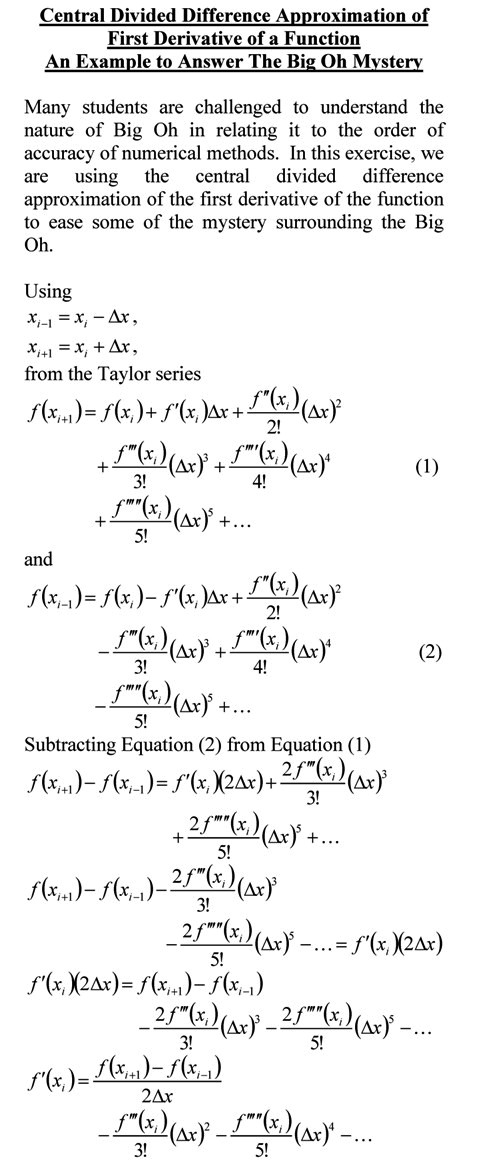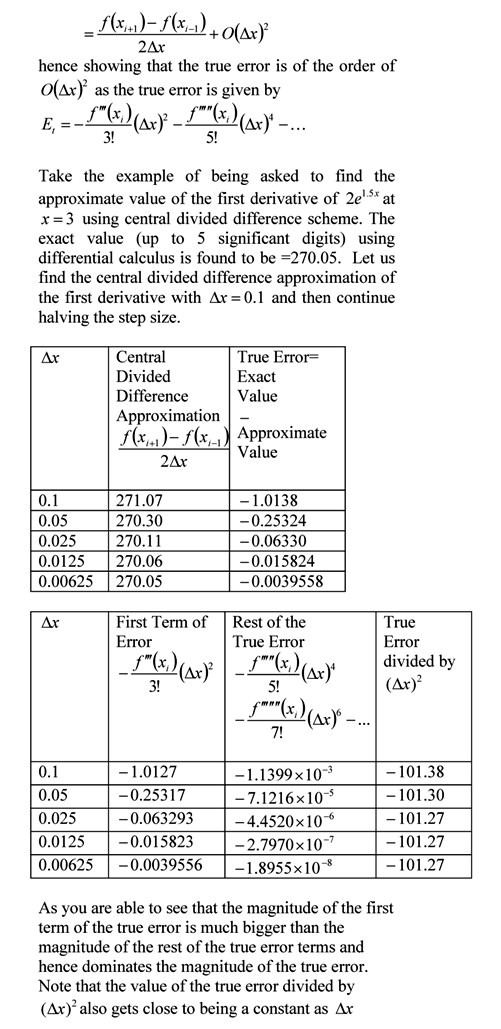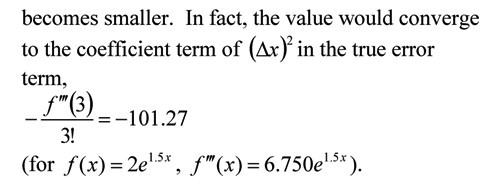You can visit the above example by opening a pdf file.

This post is brought to you by Holistic Numerical Methods: Numerical Methods for the STEM undergraduate at http://nm.MathForCollege.com, the textbook on Numerical Methods with Applications available from the lulu storefront, the textbook on Introduction to Programming Concepts Using MATLAB, and the YouTube video lectures available at http://nm.MathForCollege.com/videos.  Subscribe to the blog via a reader or email to stay updated with this blog. Let the information follow you.

## Order of accuracy of central divided difference scheme for first derivative of a function of one variable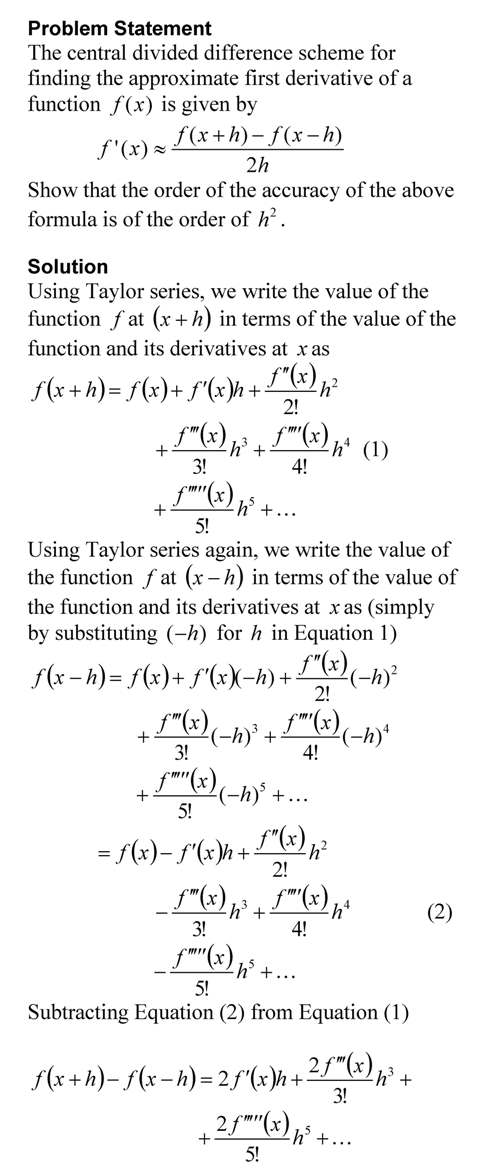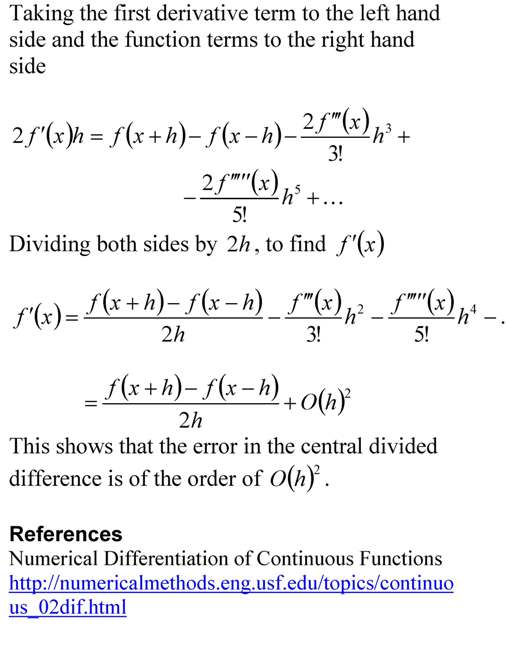This post is brought to you by
Holistic Numerical Methods: Numerical Methods for the STEM undergraduate at http://nm.mathforcollege.com, the textbook on Numerical Methods with Applications available from the lulu storefront, the textbook on Introduction to Programming Concepts Using MATLAB, and the YouTube video lectures available at http://nm.mathforcollege.com/videos.  Subscribe to the blog via a reader or email to stay updated with this blog. Let the information follow you.

## A problem using central divided difference error order

This is a problem I asked in the first examination of my Numerical Methods course in Spring 2009.   The question is that if one gives you an approximate value of the derivative of a function at a certain point using the central divided difference formula for two different step sizes, would you be able to find a better estimate of the derivative?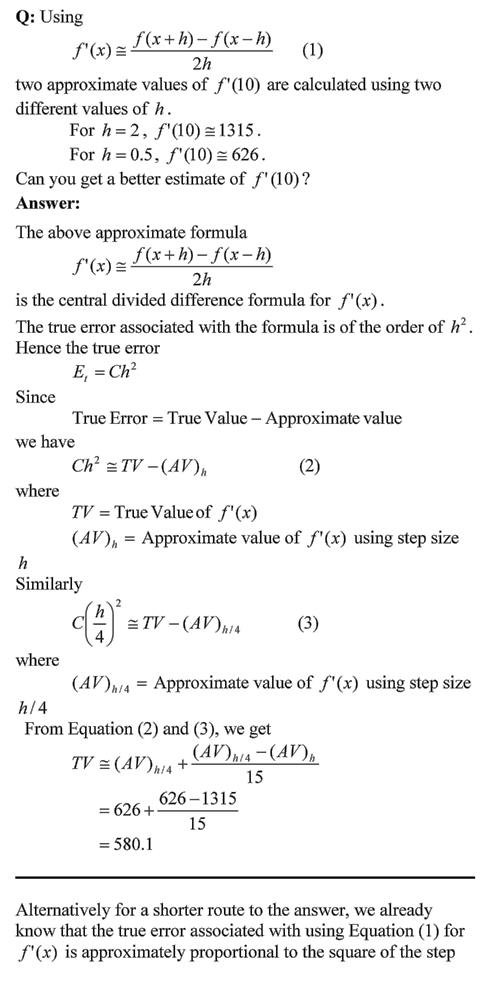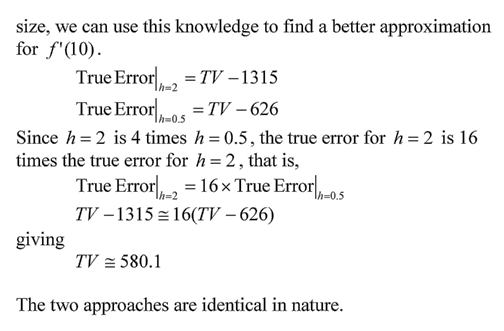This post is brought to you by Holistic Numerical Methods: Numerical Methods for the STEM undergraduate at http://nm.mathforcollege.com, the textbook on Numerical Methods with Applications available from the lulu storefront, and the YouTube video lectures available at http://www.youtube.com/numericalmethodsguy.